## How to Extract Date or Time from Datetime in Excel

Are you tired of trying to extract date or time from datetime values in Excel? This post will show you how it’s done. Whether you need to separate the date component, the time component, or both, this guide has you covered. You’ll learn how to use formulas and functions to easily extract date or time from datetime values in Excel.

This post will guide you how to extract time from a data and time value with a formula in Excel. How do I extract time from a date-time number in Excel 2013/2016. How to extract hour only from the date time format in Excel.

## 1. Extract Date from Datetime

To extract or get the date from a datetime value in one cell in Excel, you can use the following formula:

``=TEXT(datetime,"MM/DD/YYYY").``

You need to replace “`datetime`” with the cell reference that contains the datetime value. This formula converts the datetime value to text in the desired date format (`MM/DD/YYYY `in this case).

The result will be the date part of the datetime value, displayed as text string. If you need to use the result in further calculations, you can convert the result back to a date value by using the “DATEVALUE” function.

``=DATEVALUE(B2)``

You can also use the “=INT()” function to convert a date and time to a date:

=INT(A2)

This function will return the date portion of the date and time value as a serial number, which can be formatted as a date using the “Number Format” option in the “Home” tab of the Excel ribbon.

## 2. Extract Date from Text String

To extract the date from a text string in Excel, you can use the following formula:

``=MID(\$A1,SEARCH("/??/",\$A1,1)-2,10)``

where A1 is the cell that contains the text string.

This formula uses the SEARCH function to locate the position of the date part in the string and the MID function to extract the date part of the string.

## 3. Extract Year from Date

If you wish to extract the year part from a date value in Excel, you can use the YEAR function.

The YEAR function takes a date as an argument and returns the year component of the date, as a number.

For example, if cell `A1 `contains the date “`02/08/2022`“, you can extract the year using the following formula:

``=YEAR(A1)``

This formula will return the year “`2022`“.

## 4. Concatenate Date and Time

If you want to concatenate date and time in Microsoft Excel, you can use the “&” operator in combination with TEXT function to join the values in separate cells into one cell.

The Steps are as below:

Step 1: Enter the following formula in a empty cell:

``=TEXT(A2,"MM/DD/YYYY") & " " & TEXT(B2,"HH:MM:SS")``
• Replace A2 and B2 with the cells that contain your date and time, respectively.
• The formula uses the TEXT function to format the date and time values, and then joins them with a space in between.

Step 2: Press Enter to see the result of the formula.

Step 3: If you want to copy the result to multiple cells, simply select the cell with the formula, and then drag the fill handle to the cells you want to copy the formula to.

Your date and time values should now be combined into one cell in the format of “MM/DD/YYYY HH:MM:SS“.

## 5. Extract Time from Date and Time

If you want to extract time from a date and time in Excel, you can use a formula based on the TIME function, The HOUR function, the MINUTE function, and the SECOND function. For example, you want to get time value from a date and time value in Cell B1, you can write down the below formula.

``=TIME(HOUR(B1),MINUTE(B1),SECOND(B1))``

Step 1: Type this formula into a blank cell C1 and press Enter key.

Step 2: Then you need to right click on the Cell C1, and select Format Cell from the pop-up menu list. The Format Cells dialog will open.

Step 3: switch to Number tab, and click Custom from the Category list. And select one type as: h:mm:ss. Click OK button.

#4 let’s see the result.

## 6. Extract Hour only from Date and Time

If you want to extract hour only, you just need to use the HOUR function. Like this:

``=HOUR(B1)``

If you want to extract minute only from a date and time value, you just need to use the MINUTE function. Like this:

``=MINUTE(B1)``

If you want to extract second only from a date and time value, you just need to use the SECOND function. Like this:

``=SECOND(B1)``

## 7. Split Date and Time in Excel

If you want to split a date and time value into separate date and time values in Microsoft Excel, and you can use the above TEXT formula to Extract Date portion from date and time value.

Then you can use the below Text formula to extract the time portion of the date and time value:

``=TEXT(A2,”HH:MM:SS”)``

Alternatively, you can also use the INT function to extract the date portion of the date and time value, enter the following formula in a new cell:

``=INT(A2)``

Enter the following formula in another cell to extract the time portion:

``=A2-INT(A1)``

These formulas will return the date and time portions of the date and time value as serial numbers, which can be formatted as dates and times using the “Number Format” option in the “Home” tab of the Excel ribbon.

## 8. Convert Unix Timestamp to a Date in Excel

If you wish to convert a Unix timestamp to a date in Microsoft Excel, you can use the following TEXT formula:

``=TEXT((A1/86400)+DATE(1970,1,1),"MM/DD/YYYY HH:MM:SS")``

In this formula, “`A1`” represents the cell that contains the Unix timestamp. The Unix timestamp is divided by `86400 `to convert it to the number of days since January 1, 1970. The result is then added to the date “1/1/1970” to give the date and time corresponding to the Unix timestamp. The “TEXT()” function is used to format the result as a date and time in the “`MM/DD/YYYY HH:MM:SS`” format.

Note that the Unix timestamp is typically stored as an integer value representing the number of seconds since January 1, 1970, so you may need to divide the value in the cell by 86400 to convert it to a number of days.

## 9. If function with dates

The IF function in Microsoft Excel can be used with dates to perform conditional tests based on the values of dates. Here’s the general syntax for using the IF function with dates:

``=IF(condition, [value_if_true], [value_if_false])``

In this syntax, “`condition`” is the logical test that you want to perform, `[value_if_true] `is the value that will be returned if the condition is true, and `[value_if_false]` is the value that will be returned if the condition is false.

Here’s an example of using the IF function with dates:

``=IF(A1>B1, "A1 is later", "B1 is later")``

In this example, the condition “`A1>B1`” checks if the date in cell A1 is later than the date in cell B1. If the condition is true, the result will be “`A1 is later`“, and if the condition is false, the result will be “`B1 is later`“.

Note: When working with dates in Excel, it’s important to ensure that the dates are stored as dates and not as text. You can format a cell as a date by selecting it, right-clicking, selecting “Format Cells“, and then selecting the “Date” category under “Number“. From the available date formats, select the one you want to use and click “OK“.

## 10. Time Calculations in Excel

You can perform various calculations with times to get the result in terms of hours, minutes, or seconds. Here are a few examples of time calculations in Excel:

``=A1+B1``

In this example, the two times in cells A1 and B1 are added together to give the result in the same time format.

Subtract one time from another:

``=A1-B1``

In this example, the time in cell B1 is subtracted from the time in cell A1 to give the result in terms of a time.

Calculate the difference between two times in hours:

``=(A1-B1)*24``

In this example, the difference between the two times is calculated in terms of hours by multiplying the result of the subtraction by 24.

Calculate the difference between two times in minutes:

``=(A1-B1)*1440``

In this example, the difference between the two times is calculated in terms of minutes by multiplying the result of the subtraction by 1440 (24 hours * 60 minutes).

## 11. Conclusion

In conclusion, extracting dates or times from datetime values in Microsoft Excel is very useful for data analysis and manipulation. Whether you need to separate dates and times for the purpose of sorting or calculating, or just to display the information in a different format, there are several ways to achieve it. Excel offers a range of options for extracting date or time information from datetime values.

## 12. Related Functions

• Excel TIME function
The Excel TIME function returns a date in serial number format for a particular time (the hour, minute and second).The syntax of the TIME function is as below:= TIME(hour, minute, second)…
• Excel HOUR Function
The Excel HOUR function returns the hour of a time value. Or returns an integer value that represent the hour component of a given time. The syntax of the HOUR function is as below:=HOUR (serial_number) …
• Excel MINUTE Function
The Excel MINUTE function returns the minutes of a time value. And the minutes is an integer number from 0 to 59. The syntax of the MINUTE function is as below:=MINUTE (serial_number)…
• Excel SECOND Function
The Excel SECOND function returns the seconds of a time value. Or returns an integer value that represent the second component of a given excel time. And the return value is between 0-59.The syntax of the SECOND function is as below:=SECOND (serial_number)…

## Add Minutes to Time in Excel

Adding whole hours or decimal minutes to a given time in Excel is a fairly simple process. You can do this by using the Start_time+TIME(0,minutes,0) and Start_time+minutes/1440 functions. In this add minutes to time guide, we will show you how to do this.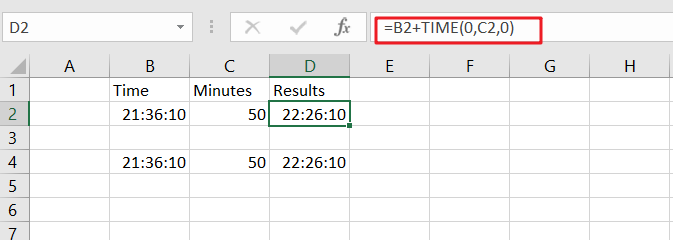If you want to add whole minutes to a given time, you can use the following formula:

`=Start_time+TIME(0, minutes,0)`
• Start_time: the time that you want to add minutes to
• Minutes: the minutes that you wish to add

This function returns a time formatted string.

Cell C2 shows the number of minutes you want to add, while Cell D2 displays a formula that will calculate how many more cells need changing.

`=B2+TIME(0,C2,0)`

Or

`=B4+C4/1440`

To see the result, press Enter key.### Explanation

The TIME function is a great way to convert decimal numbers into an appropriate time format. Here, we change the number of minutes from 50 (which converts it into PM) and then add:00 before adding another set amount for minutes-in, in this case, 0 because there aren’t any other digits available on our keyboard!

Note: To subtract the minutes from a time period, you need to use this formula.

`= MOD(Start_time-TIME(0, minutes,0),1)`

For Instance, Cell B2 shows time, and cell C2 shows the number of minutes you want to subtract. If you want to subtract a number of minutes, use the below formula:

`=MOD(B2-TIME(0,C2,0),1)`

To see the result, hit Enter key.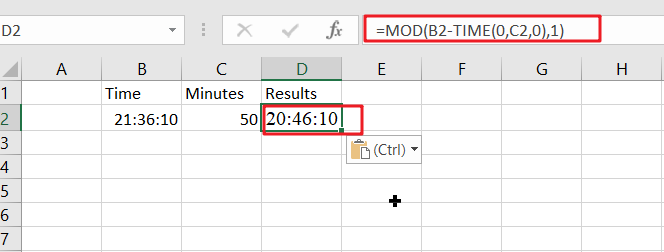The MOD (modulus) function is an important tool for financial professionals. It can be used to flip negative numbers into positive ones yield accurate results in most cases!

## Add decimal Minutes to time

Generic formula:

`=Start_time+ minutes /1440`
• Start_time: the time that you wish to add minutes to.
• minutes: the minutes that you wish to add to start_time.

The value should be returned in time format.

For instance, cell B2 contains the time value, and cell C2 displays the number of minutes you want to add. Please see the below formula for this purpose.

`=B2+C2/1440`

To see the result hit Enter key.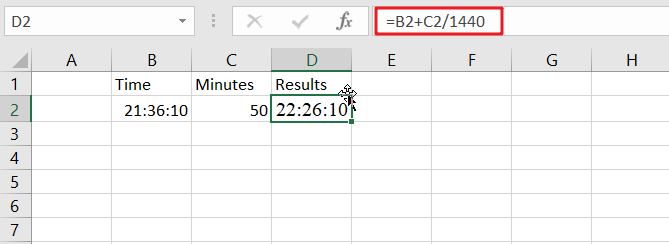24 hours in a day, and one minutes is 1/1440th of the whole duration.

Note: Subtracting the minutes from time is easy with this formula.

`=MOD(Start_time- minutes /1440,1)`

The number of minutes you want to subtract is displayed in cell C2, and time is displayed in B2. To find the correct formula, please use this simple equation:

`=MOD(B2-C2/1440,1)`

To see the result hit Enter key.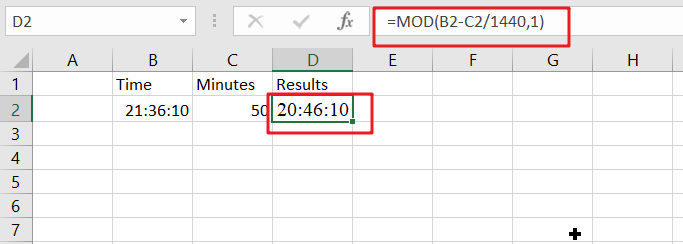When Excel detects negative values in a cell, it converts them to positive with the MOD function. This means you can just flip your negatives around and get an accurate time reflecting how long something takes!

### Related Functions

• Excel TIME function
The Excel TIME function returns a date in serial number format for a particular time (the hour, minute and second).The syntax of the TIME function is as below:= TIME(hour, minute, second)…
• Excel MOD function
the Excel MOD function returns the remainder of two numbers after division. So you can use the MOD function to get the remainder after a number is divided by a divisor in Excel. The syntax of the MOD function is as below:=MOD (number, divisor)….

## Add Hours To Time in Excel

Adding whole hours or decimal hours to a given time in Excel is a fairly simple process. You can do this by using the Start_time+TIME(hours,0,0) and Start_time+hours/24 functions. In this add hours to time guide, we will show you how to do this.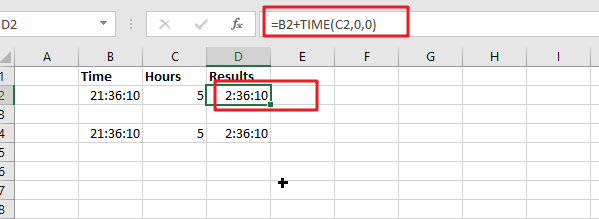If you want to add whole hours to a given time, you can use the following formula:

`=Start_time+TIME(hours,0,0)`
• `Start``_time`: the time that you want to add hours to
• `Hours`: the hours that you wish to add

This function returns a time formatted string.

Cell C2 shows the number of hours you want to add, while Cell D2 displays a formula that will calculate how many more cells need changing.

`=B2+TIME(C2,0,0)`

Or

`=B4+C4/24`

To see the result, press Enter key.### Explanation

The TIME function is a great way to convert decimal numbers into an appropriate time format. Here, we change the number of hours from 5 (which converts it into PM) and then add:00 before adding another set amount for minutes-in, in this case, 0 because there aren’t any other digits available on our keyboard!

Note: To subtract the hours from a time period, you need to use this formula.

`= MOD(Start_time-TIME(hours,0,0),1)`

For Instance, Cell B2 shows time, and cell C2 shows the number of houses you want to subtract. If you want to subtract a number of hours, use the below formula:

`=MOD(B2-TIME(C2,0,0),1)`

To see the result, hit Enter key.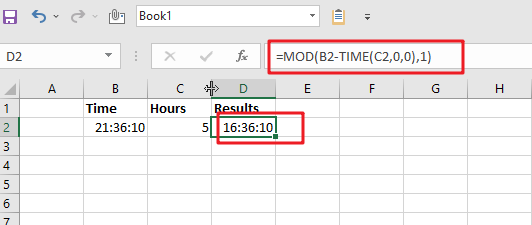The MOD (modulus) function is an important tool for financial professionals. It can be used to flip negative numbers into positive ones yield accurate results in most cases!

## Add decimal hours to time

Generic formula:

`=Start_time+hours/24`
• `Start_time`: the time that you wish to add hours to.
• `Hours`: the hours that you wish to add to start_time.

The value should be returned in time format.

For instance, cell B2 contains the time value, and cell C2 displays the number of hours you want to add. Please see the below formula for this purpose.

`=B2+C2/24`

To see the result hit Enter key.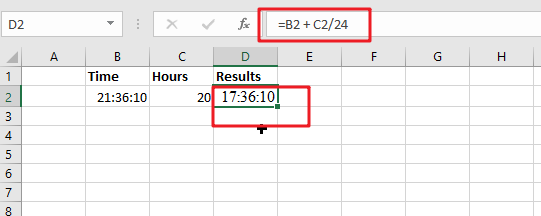24 hours in a day, and one hour is 1/24th of the whole duration.

Note: Subtracting the hours from time is easy with this formula.

`=MOD(Start_time-hours/24,1)`

The number of hours you want to subtract is displayed in cell C2, and time is displayed in B2. To find the correct formula, please use this simple equation:

`=MOD(B2-C2/24,1)`

To see the result hit Enter key.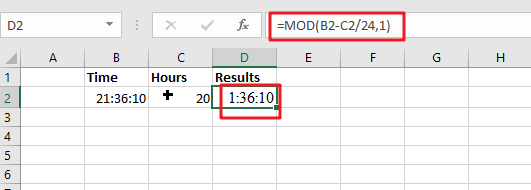When Excel detects negative values in a cell, it converts them to positive with the MOD function. This means you can just flip your negatives around and get an accurate time reflecting how long something takes!

Note:

1. The number of hours when using the Start_time + hours / 24 formula can be a decimal number. See screenshot: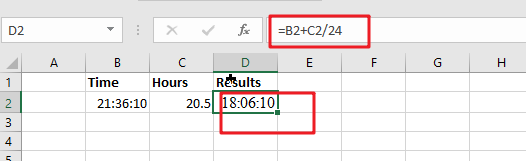2. If the result is over 24 hours, then under the Formate cell’s Number tab, go to the Custom section and format the result as [h]:mm:ss.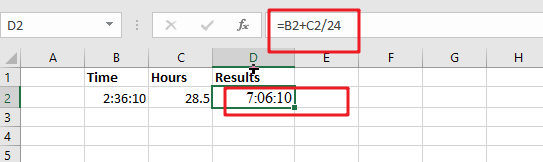### Related Functions

• Excel TIME function
The Excel TIME function returns a date in serial number format for a particular time (the hour, minute and second).The syntax of the TIME function is as below:= TIME(hour, minute, second)…
• Excel MOD function
the Excel MOD function returns the remainder of two numbers after division. So you can use the MOD function to get the remainder after a number is divided by a divisor in Excel. The syntax of the MOD function is as below:=MOD (number, divisor)….

## How to Compare Time Difference with Certain Time in Excel

Sometimes we need to count time difference when participating a contest or an exam, and if the time difference (between start time and end time) is greater than certain time, participants are failed in the contest. So, in this situation we need to know the method to compare time difference with certain time. This article will introduce you a quick method to fix this issue.

Precondition:

Prepare a table record the start time and end time of a contest. And if time difference is greater than 2 hours, participators fail the contest, on the other side, if time difference is less than 2 hours, they pass the contest. We need to enter PASS or FAIL in column C.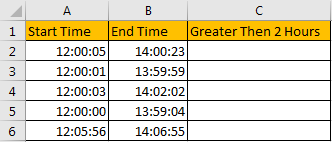## Method: Compare Time Difference with Certain Time in Excel

Step 1: In C2 enter the formula =IF(B2 > A2 + TIME(2,0,0),”FAIL”,”PASS”). In this formula, if B2 is greater than A2+2 hours, then formula returns FAIL, otherwise it returns PASS.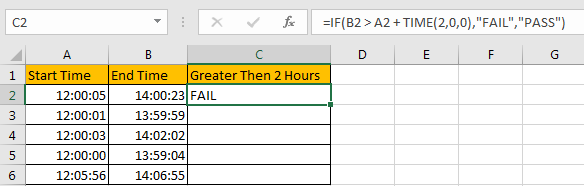Step 2: Drag the fill handle down to fill B3:B6. Verify that B3:B6 are filled with FAIL or PASS.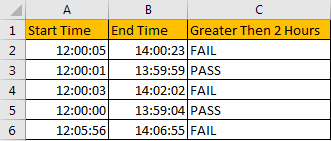We can see that in this example, only two people pass the contest.

Comment:

1. As TIME(HOUR,MINUTE,SECOND), so we can change hour/minute/second values per our real demands. If we want compare time difference with 2 hour 5 minutes 10 seconds, we can update the formula as =IF(B2 > A2 + TIME(2,5,10),”FAIL”,”PASS”).

### Related Functions

• Excel TIME function
The Excel TIME function returns a date in serial number format for a particular time (the hour, minute and second).The syntax of the TIME function is as below:= TIME(hour, minute, second)…
• Excel IF function
The Excel IF function perform a logical test to return one value if the condition is TRUE and return another value if the condition is FALSE. The IF function is a build-in function in Microsoft Excel and it is categorized as a Logical Function.The syntax of the IF function is as below:= IF (condition, [true_value], [false_value])….

## How to Convert Text to Time in Excel

This post will guide you how to convert time string to time in Excel. Assuming that you have a list of text string in your worksheet, and you wish to convert these time string to standard time format, how to do it.

You need to use an formula based on the TIMEVALUE function, the LEFT function, the LEN function and the RIGHT function. Like below:

`=TIMEVALUE(LEFT(B1,LEN(B1)-2)&":"&RIGHT(B1,2))`

Type this formula into a blank cell, and press Enter key on your keyboard to apply it. and drag the AutoFill Handle to other cells to apply this formula.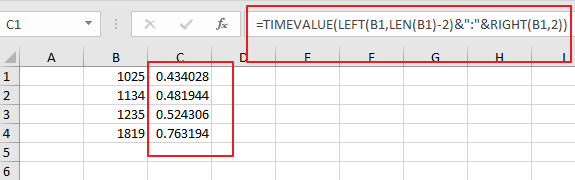Then you need to keep these cells selected, and right click on it, select Format Cells from the context menu list and the Format Cell Dialog will open.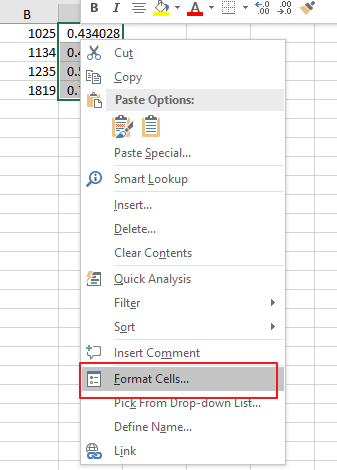Switch to Number tab under Format Cells dialog box, and select Time category from the Category list, and select one time type as you need. click Ok button.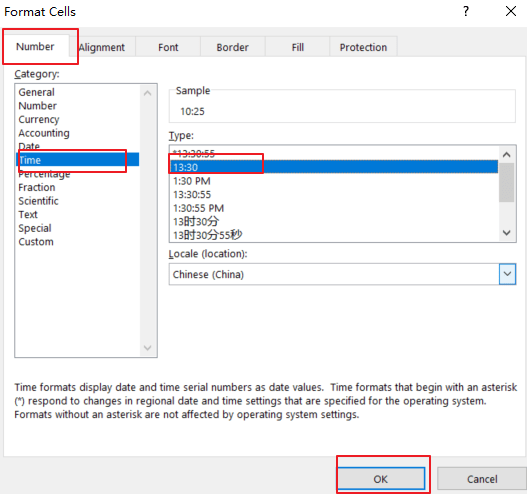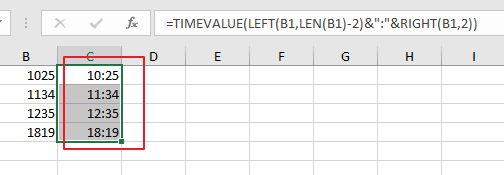If you want to convert date and time string to a data time format in Excel, and you can use the following formula based on the DATE function, the LEFT function, the MID function, the Time Function and the RIGHT function. Like below:

`=DATE(LEFT(B1,4),MID(B1,5,2),MID(B1,7,2))+TIME(MID(B1,10,2),RIGHT(B1,2),0)`

Type this formula into a blank cell and press Enter key on your keyaboard.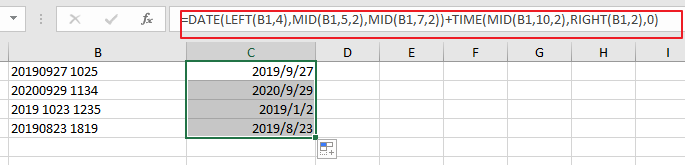Then you need to format those selected cells to a data format as you need.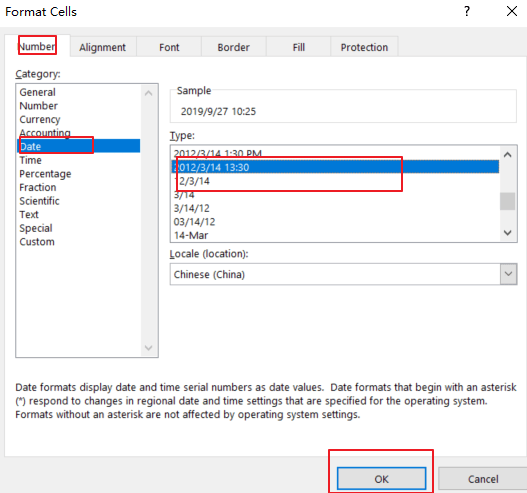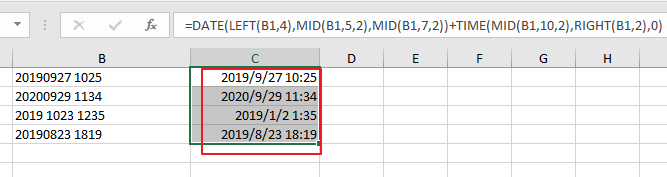### Related Functions

• Excel TIMEVALUE Function
The Excel TIMEVALUE function returns the decimal number of the time represented by a text string. so it will convert a time represented by a text string into an Excel time value.The syntax of the TIMEVALUE function is as below:=TIMEVALUE (time_text)…
• Excel MID function
The Excel MID function returns a substring from a text string at the position that you specify.The syntax of the MID function is as below:= MID (text, start_num, num_chars)…
• Excel LEFT function
The Excel LEFT function returns a substring (a specified number of the characters) from a text string, starting from the leftmost character.The LEFT function is a build-in function in Microsoft Excel and it is categorized as a Text Function.The syntax of the LEFT function is as below:= LEFT(text,[num_chars])…
• Excel RIGHT function
The Excel RIGHT function returns a substring (a specified number of the characters) from a text string, starting from the rightmost character.The syntax of the RIGHT function is as below:= RIGHT (text,[num_chars])…
• Excel TIME function
The Excel TIME function returns a date in serial number format for a particular time (the hour, minute and second).The syntax of the TIME function is as below:= TIME(hour, minute, second)…

## How to Extract Time From A Date And Time in Excel

This post will guide you how to extract time portion from a date and time in Excel. How do I remove date on Date/Time Stamp with a formula in Excel. How to remove date from a date and time value with find and replace feature in Excel 2013/2016.

Assuming that you have a list of data in range B1:B4 in which contain date and time stamps. You want to pull out the time portion only from the given cells in Excel. This post will show you two methods to remote the date from a cell with date and time in it.

## Method1: Extract Time from a Date and Time Using Formula

You can remove the date portion from a date and time stamps by using the TIME function, HOUR function, MINUTE function, and SECOND function. Just see the example below, removing the date portion from those values in range B1:B4 using the following formula:

`=TIME(HOUR(B1),MINUTE(B1),SECOND(B1))`

Type this formula into a blank cell and press Enter key on the keyboard, and drag the AutoFill Handle down to other cells to apply this formula.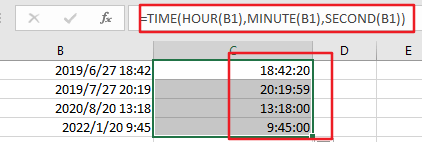Let’s see how this formula works:

The HOUR, MINUTE and SECOND functions are used to extract values for the Hour, Minute and Second portions. Then the TIME function will format those values as a Time stamp.

You can also use another formula based on the INT function to achieve the same result. Like this:

`=B1-INT(B1)`

Type this formula into a blank cell and press Enter key, then drag the AutoFill handle over to the other cells that you want to apply the same formula. The serial number of time portion would be extracted. Then you need to reformat those cells to a time format, it will allow the fraction to show as a normal Time value.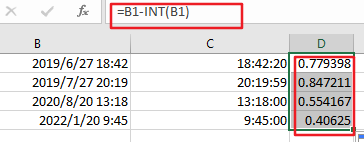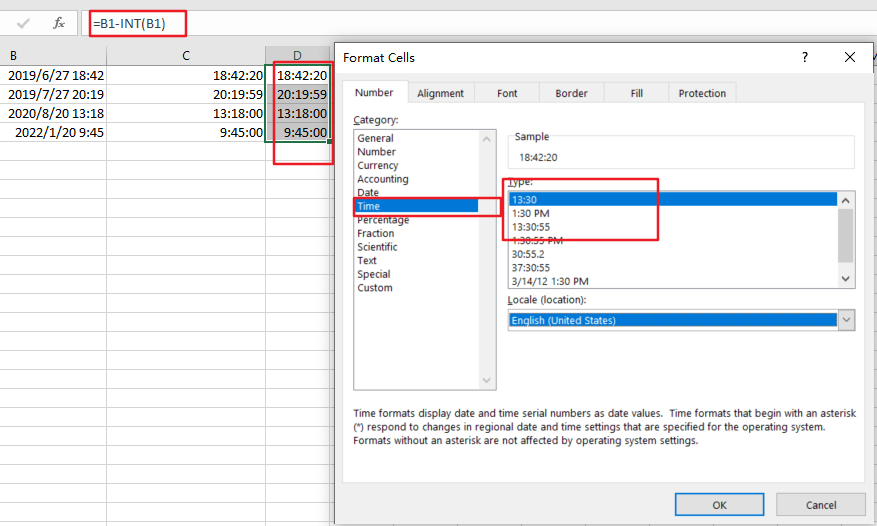## Method2: Extract Time from a Date and Time Using Find and Replace

You can also use Find and Replace feature to extract time portion from a date and time stamp in Excel. Just do the following steps:

Step1: select the range of cells that contain date and time values.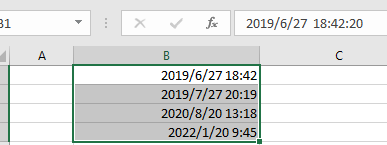Step2: right click on the selected cells, and choose Format Cells from the dropdown menu list. And the Format Cells dialog will open.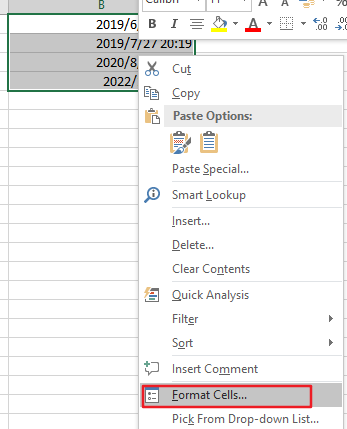Step3: select General category under the Category drop list, it will change the normal date and time forma to general format. click Ok button to return to the current worksheet.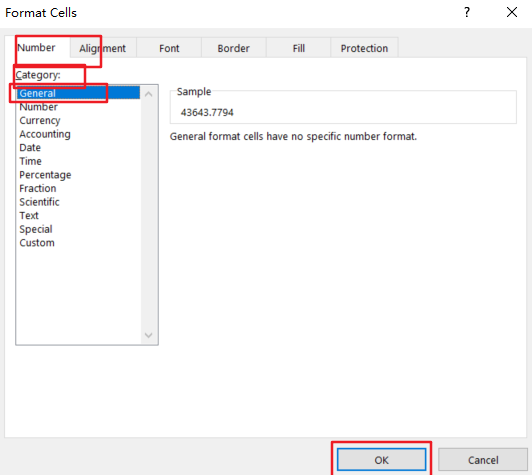Step4: go to Home tab in the Excel Ribbon, click Find & Select command under Editing group, and choose Replace from the dropdown list. And the Find and Replace dialog will open.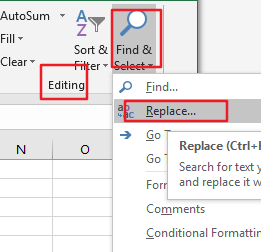Step5: enter the pattern *. Into the Find what text box, and enter the another pattern . into the Replace with text box in the Find and Replace dialog box.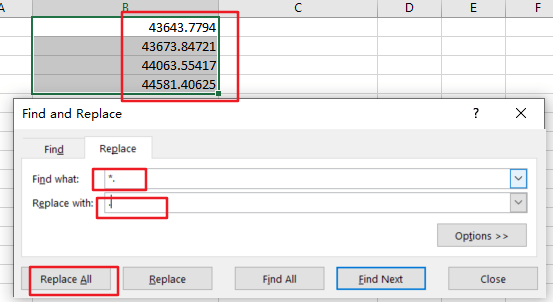Step6: click Replace All button, click close button to back to the current worksheet. You would get the serial number of the time portion for all the selected date and time values.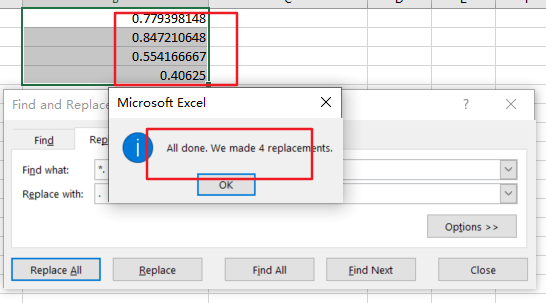Step7: keep those values selected, and right click on it, and select Format cells, and choose Time category under the Category drop list. And select one type in the Type list box, click Ok button.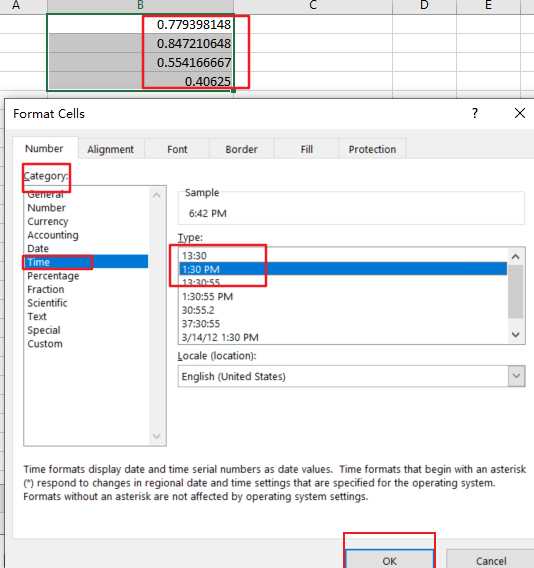Step8: you would see that all time portions have been extracted.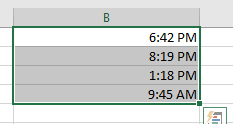### Related Functions

• Excel TIME function
The Excel TIME function returns a date in serial number format for a particular time (the hour, minute and second).The syntax of the TIME function is as below:= TIME(hour, minute, second)…
• Excel HOUR Function
The Excel HOUR function returns the hour of a time value. Or returns an integer value that represent the hour component of a given time. The syntax of the HOUR function is as below:=HOUR (serial_number) …
• Excel MINUTE Function
The Excel MINUTE function returns the minutes of a time value. And the minutes is an integer number from 0 to 59. The syntax of the MINUTE function is as below:=MINUTE (serial_number)…
• Excel SECOND Function
The Excel SECOND function returns the seconds of a time value. Or returns an integer value that represent the second component of a given excel time. And the return value is between 0-59.The syntax of the SECOND function is as below:=SECOND (serial_number)…

## Adding Hours, Minutes, or Seconds to a Date and Time in Excel

This post will guide you how to add hours, minutes, or seconds to a given date and time in a cell in Excel. How do I add hours, minutes or seconds to a time with a formula in Excel. How to add hours to a date and time in Excel. How do I add 15 minutes to a time in excel.

## Add Hours to a Date and Time

If you want to add 2 hours to a specific date and time in one cell, you can use the following formula to achieve the result.

`=B1+ 2/24 `

You need to type this formula into a blank cell and then press Enter key in your keyboard, and drag the AutoFill Handle over other cells to apply this formula to add 2 hours for other dates.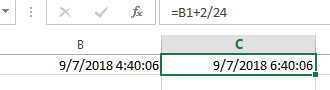This formula will return a serial number, so you need to format the serial number as the standard date and time format.

## Add Minutes to a Date and Time

If you want to add 15 minutes to a date and time in one cell, you can use the following formula:

`=B1 + 15/1440`

## Add Seconds to a Date and Time

If you want to add 1 second to a date and time in one cell, you can use the following formula:

`=B1 + 1/18640`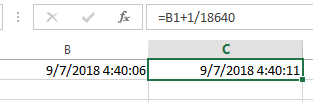You can also create another formula based on the TIME function to achieve the same result.
For example, you want to add 2 hours, 15 minutes, 1 second to a data and time value in Cell B1, you can use the following formula:

`=B1 + TIME(2,15,1)`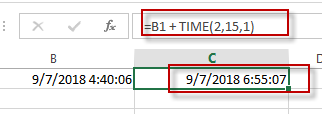## Excel TIME Function

This post will guide you how to use Excel TIME function with syntax and examples in Microsoft excel.

### Description

The Excel TIME function returns a date in serial number format for a particular time (the hour, minute and second).

The serial number returned by TIME function is a value between 0 and 0.99988426. And the result of 0 represents 12:00:00 AM and the result of 0.99988426 represents 11:59:59 PM.

So this function can be used to create a time with hours, minutes, and seconds. And it also will return a decimal number representing a particular time in excel.

The TIME function is a build-in function in Microsoft Excel and it is categorized as a DATE and TIME Function.
The TIME function is available in Excel 2016, Excel 2013, Excel 2010, Excel 2007, Excel 2003, Excel XP, Excel 2000, Excel 2011 for Mac.

### Syntax

The syntax of the TIME function is as below:

`= TIME(hour, minute, second)`

Where the TIME function arguments are:

• Hour -This is a required argument. A number from 0 (zero) to 32767 representing the hour. If the value is greater than 23 and then it will be divided by 24 and the remainder will be treated as the hour value. For example, the TIME(28,0,0) is equal to TIME(4,0,0).
• Minute – This is a required argument. A number from 0 to 32767 representing the minute. If the minute value is greater than 59 and it will be converted to hours and minutes (it means that every 60 minutes will add one hour to hour value). For example, TIME(1,75,0) = TIME(2,15,0).
• Second – This is a required argument. A number from 0 to 32767 representing the second. If the second value is greater than 59 and it will be converted to hours, minutes, and seconds (it means that every 60 seconds will add 1 minute to minute value). For example, TIME(1,20,75) = TIME(1,21,15) .

Excel TIME Function Examples
The below examples will show you how to use Excel TIME function to create a decimal number representing a particular time.

#1 =TIME(A2,B2,C2)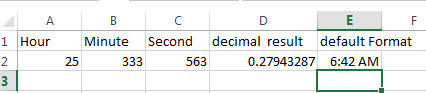## How to Group Time by Hour or Minute

This post will guide you how to group time by hours intervals or group time by minute intervals in excel. How to group time by 3 hours using excel formula? How to group time by 15 minutes with formula in excel.

## Group Time by Hour or Minute

If you have a list of times in your worksheet and wonder if you want to group them into intervals such as 3 hours, one hour or 15 minutes.  How to quickly group time in excel? You can use a rounding function named as FLOOR in excel.  This function can be used to round the times based on the significance that you specified.

You can use the FLOOR function to create a generic formula as follows:

`=FLOOR(number,significance)`

Number – the number that you want to round down

Significance – the multiple of significance that you want to round a number to.

If the time is 16:00 PM and the significance value as “3:00” , then the FLOOR function should be round the time value down to the 3 hours intervals, and it will return as: 15:00 PM.

Suppose you need to group times in range A2:A6 by 3 hours, then you can write down the following formula:

`=FLOOR(A2,”3:00”)`

1# you can enter this formula into cell B2, then press Enter key.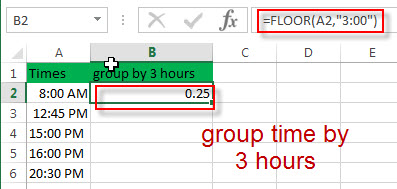2# select cell B1, right click on it, select Format Cells…, switch to Number tab, choose Time under Category list. Then click “OK” button.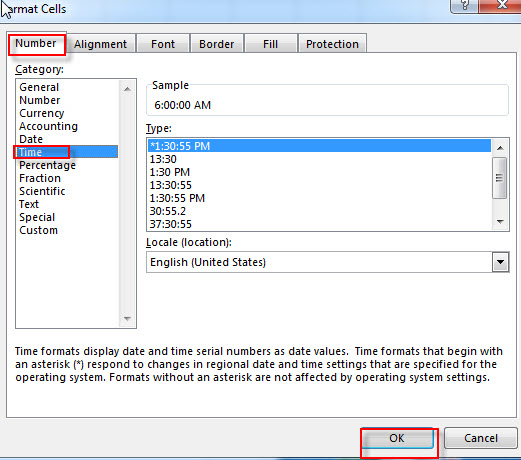3# let’s see the result.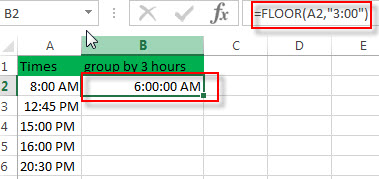4# drag the AutoFill handle down to apply this formula to other cells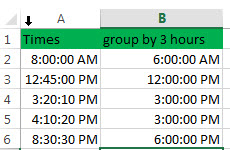If you want to group time by 15 minutes in your list of times in range A2:A6, then you use the following formula:

`=FLOOR(A2, “0.05”)`

Or

`=FLOOR(A2,TIME(0,15,0))`

You can enter this formula into Cell B1, then press Enter.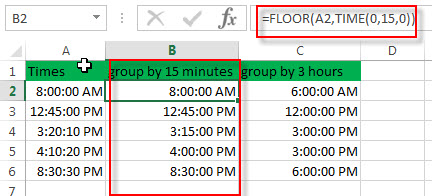## Group time with pivot table

You can also use pivot table to group times by the hour, but it only be able to group the times by 1 hour increments. It can’t group into other specific time intervals, such as: 15 minutes, or 3 hours.
1# Go to “INSERT” Tab, then click Tables -> PivotTable. Then Create PivotTable window will appear.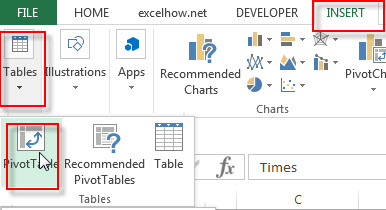2# select a table or range A1:A6, then click “OK” button.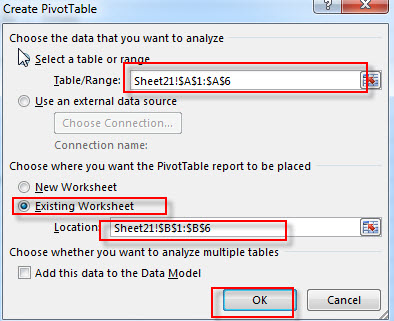3# Choose Times field to the Rows area.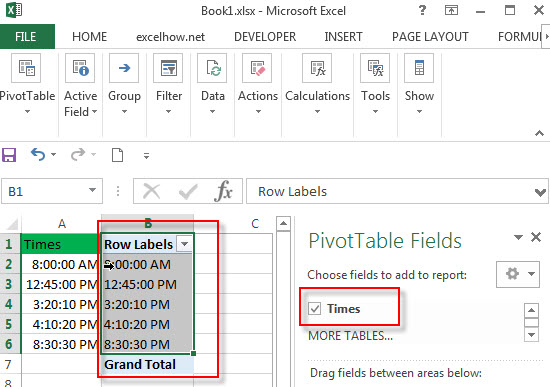4# right click on any cell in the Rows area and choose Group…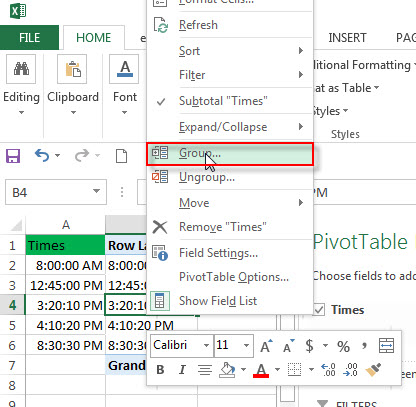5# choose Hours from Grouping window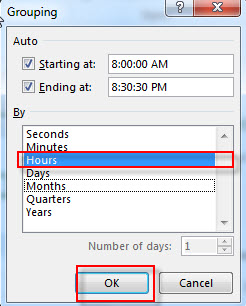6# you should see that the times have been grouped by 1 hour.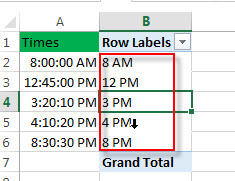### Related Functions

• Excel FLOOR function
The Excel FLOOR function returns a number rounded down to the nearest multiple of significance. So it will return a rounded number.The syntax of the FLOOR function is as below:= FLOOR (number, significance)…
• Excel TIME function
The Excel TIME function returns a date in serial number format for a particular time (the hour, minute and second).The syntax of the TIME function is as below:= TIME(hour, minute, second)…